## RS Aggarwal Class 7 Solutions Chapter 4 Rational Numbers Ex 4D

These Solutions are part of RS Aggarwal Solutions Class 7. Here we have given RS Aggarwal Solutions Class 7 Chapter 4 Rational Numbers Ex 4D.

Other Exercises

Question 1.
Solution:
(i) Additive inverse of 5 = -5
(ii) Additive inverse of -9 = – (-9) = 9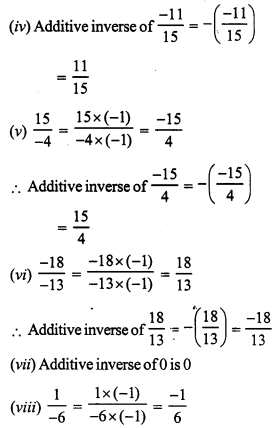Question 2.
Solution: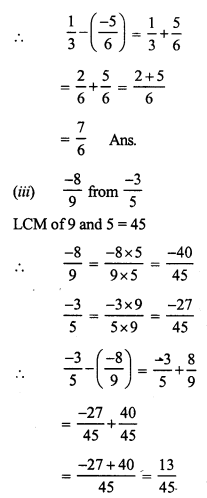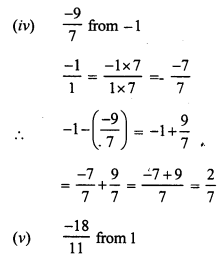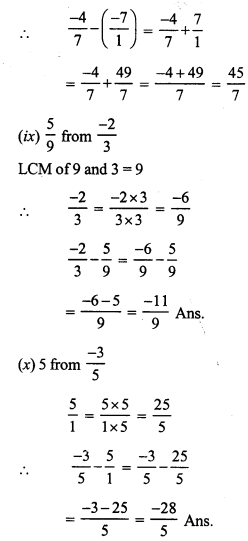Question 3.
Solution: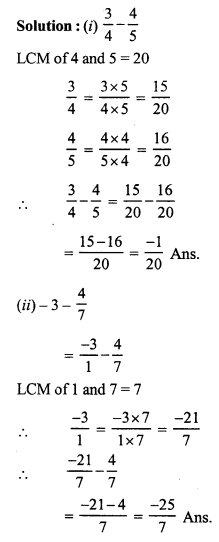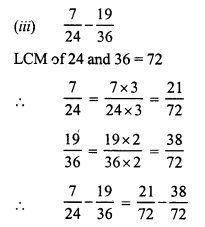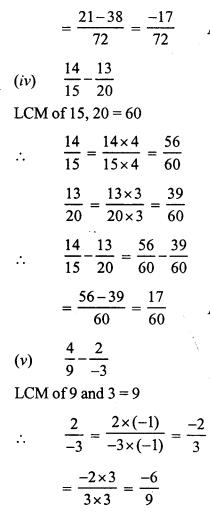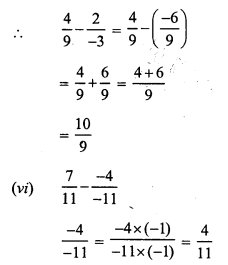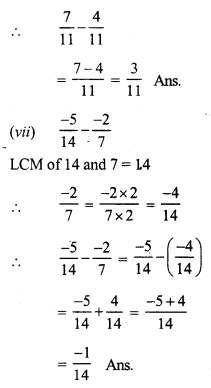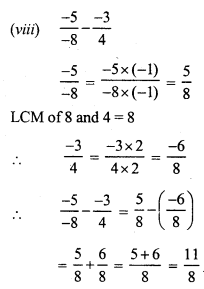Question 4.
Solution: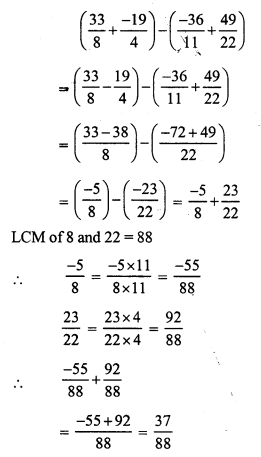Question 5.
Solution: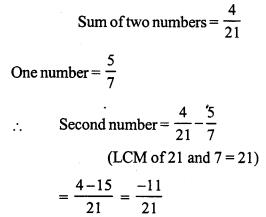Question 6.
Solution: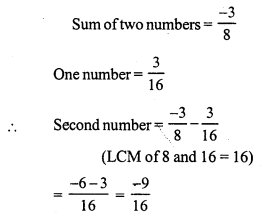Question 7.
Solution: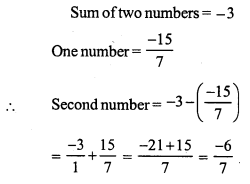Question 8.
Solution: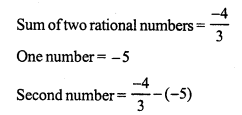Question 9.
Solution: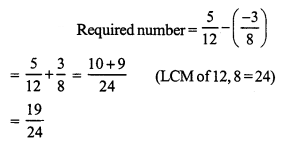Question 10.
Solution:Question 11.
Solution: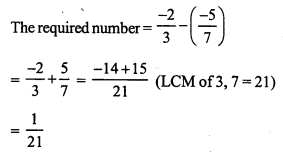Question 12.
Solution:
The required number = $$\frac { -1 }{ 1 }$$ – $$\frac { 2 }{ 9 }$$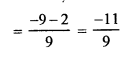Question 13.
Solution:Question 14.
Solution: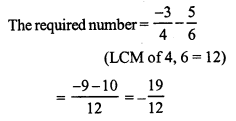Question 15.
Solution: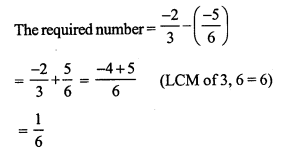Question 16.
Solution: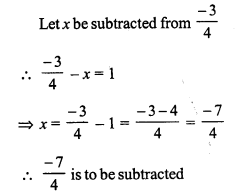Hope given RS Aggarwal Solutions Class 7 Chapter 4 Rational Numbers Ex 4D are helpful to complete your math homework.

If you have any doubts, please comment below. Learn Insta try to provide online math tutoring for you.